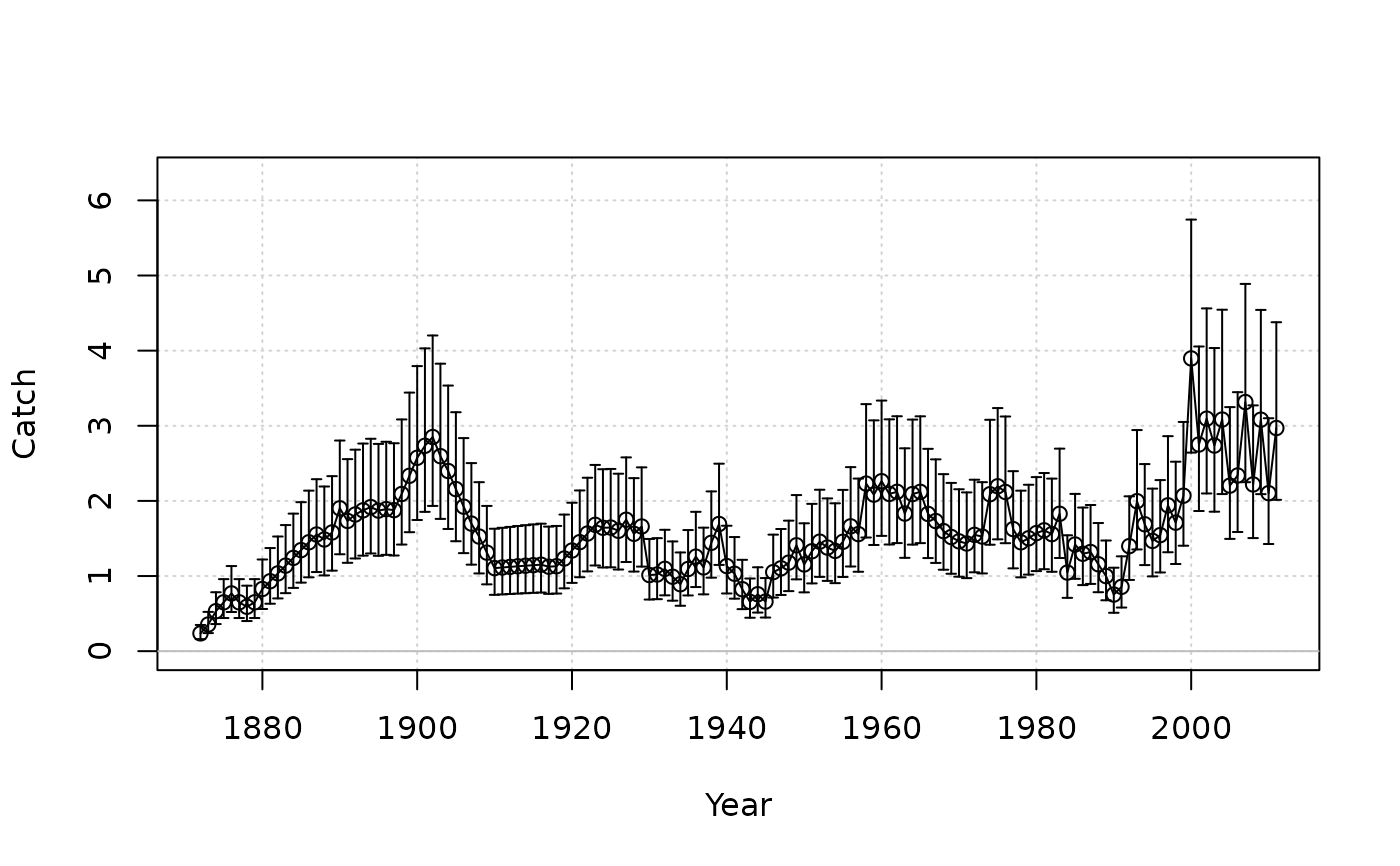Plot time series of observed (with lognormally-distributed error bars) vs. predicted data.

plot_timeseries(
Year,
obs,
fit = NULL,
obs_CV = NULL,
obs_CV_CI = 0.95,
obs_upper = NULL,
obs_lower = NULL,
obs_ind_blue = NULL,
fit_linewidth = 3,
fit_color = "red",
label = "Observed data"
)

## Arguments

Year

A vector of years for the data.

obs

A vector of observed data.

fit

A vector of predicted data (e.g., from an assessment model).

obs_CV

A vector of year-specific coefficient of variation in the observed data.

obs_CV_CI

The confidence interval for the error bars based for obs_CV.

obs_upper

A vector of year-specific upper bounds for the error bars of the observed data (in lieu of argument obs_CV).

obs_lower

A vector of year-specific lower bounds for the error bars of the observed data (in lieu of argument obs_CV).

obs_ind_blue

Indices of obs for which the plotted points and error bars will be blue.

fit_linewidth

Argument lwd for fitted line.

fit_color

Color of fitted line.

label

Character string that describes the data to label the y-axis.

## Value

A plot of annual observed data and predicted values from a model.

plot_residuals

Q. Huynh

## Examples

data(Red_snapper)
plot_timeseries(Red_snapper@Year, Red_snapper@Cat[1, ],
obs_CV = Red_snapper@CV_Cat, label = "Catch")